# Volume of a Pyramid – Without Calculus

Last week we looked at ways to derive the formulas for volume and surface area of a sphere, without using calculus. Let’s do the same this time for a pyramid. We’ll be seeing one method that comes very close to calculus (slicing and infinite series), and another that is fully geometrical (dissection, which we’ll do three different ways).

## Why 1/3?

The first question we’ll look at comes from Terence, in 1998:

Volume of a Pyramid

How come the volume of a pyramid is equal to 1/3 the volume of a prism of the same base area and height? Can you explain it to me?

The formula for the volume of a pyramid is, in the most general form, $$V = \frac{1}{3}Bh$$ where B is the area of the base (regardless of its shape), and h is the height from the base to the apex. This also applies to cones.

This had already been answered (a little tersely, by Doctor Rob) 5 months before, so I answered initially with a reference to that page, as well as a link to Euclid’s Elements, which uses a very different method:

A lot of students wonder about this, and we seldom bother to give a proof, because it generally is beyond the abilities of students at the level we teach the formula.

There is an explanation using infinite series in our Dr. Math archives at

Volume of a Cone or Pyramid
http://mathforum.org/dr.math/problems/swan3.30.98.html

This is rather brief, so if you need help understanding it, write back!

You may also be interested in seeing how Euclid proved it (complete with a picture, if your browser supports Java):

Book XII, Proposition 7, Euclid's Elements - David Joyce
http://aleph0.clarku.edu/~djoyce/java/elements/bookXII/propXII7.html

This shows a way to divide a triangular prism into three pyramids of equal volume; a little more work is needed to complete the proof for all pyramids and for cones, which Euclid does next.

Neither of these references is good for a beginner, so I expected a request for help in understanding them, and I was not surprised by the reply:

I do not understand the information on the Web site you sent me. Can you further explain it to me? Thanks.

So this time I expanded on both methods:

I was expecting to hear back from you. This proof is a little complicated, which is why we don't generally bother to teach it. Most students are satisfied either to trust mathematicians, or to try it out by measuring the volume of a pyramid and seeing that the formula works. It's good that you're not satisfied with that, and want to know the reasons behind what you learn.

### Slicing the pyramid

I demonstrated with a square-based pyramid, though the same reasoning applies to any base.

The first page I referred you to, at

http://mathforum.org/dr.math/problems/swan3.30.98.html

gives this calculation for the volume of a square pyramid:

V = a^2*b*[1^2 + 2^2 + 3^2 + ... + n^2]
= a^2*b*n*(n + 1)*(2*n + 1)/6  (which can be proved by induction)
= a^2*b*n^3*(1/3 + 1/[2*n] + 1/[6*n^2])

Here's what it means:

Suppose we try to build a square pyramid out of flat square boxes (whose volume we can calculate as L x W x H). It won't be perfect, but will look like a "step pyramid":

*-* ----------------------------------
**-*|----*                            ^
*/ | |*   /|----*                       |
*//  *-*   / *   /|----*                  |
*//*--------* /   / *   /|----*             |
/// |        |/   / /   / *   /|b            H
///  *--------*   / /   / /   / *             |
//*---------------* /   / /   / /              |
// |               |/   / /  a/ /               |
//  *---------------*   / /   / /                v
/*----------------------* /   / /B -----------------
/ |                      |/   / /
/  *----------------------*   / /
*-----------------------------* /
|              a              |/
*-----------------------------*
B

We can imagine that we’ve sliced a pyramid into N equal slices, and then added some material on the outside so each slice has vertical walls. Or maybe we’ve piled up layers of cake, and are going to cut off some material to make a smooth pyramid.

Let's build it so that the base of each horizontal slab is a cross-section of the pyramid we are trying to measure, so that this step pyramid is larger than the real pyramid. Say the base is B by B, so its area is B^2, and the height is H. If we have divided the height into N slabs, then the height of each slab is H/N, and the width of the K'th slab from the top is B*K/N. (Do you see why? The top slab is B/N, the next is 2B/N, ..., the bottom one is B*N/N = B.)

(Note that his a is my B, and his b is my H/N.)

You can use similar triangles to explain the widths. Then each slab’s volume is its side squared, times its height:

Then the volume of the whole thing is the sum of the volumes of the slabs:

(B*1/N)^2 * H/N + (B*2/N)^2 * H/N + ... + (B*N/N)^2 * H/N

and we can factor out (B/N)^2 * H/N from all the terms to get:

(B/N)^2 * H/N * (1^2 + 2^2 + ...+ N^2)

There is a formula for the sum of squares, which is:

1^2 + 2^2 + ...+ N^2 = N*(N + 1)*(2N + 1)/6

This formula for a sum of squares is explained in many places; I’ll have a post about several ways to prove it soon. I gave one method (because that’s what Doctor Rob suggested), in case you have learned about mathematical induction:

This can be proved by induction; that is, by showing that it is true for N = 1, and that if it is true for N, it is also true for N+1. Once that is proved, it must be true for all N. Here is a quick proof:

For N = 1, 1^2 = 1*2*3/6 is true.
If true for N, then
1^2 + ... + N^2 + (N+1)^2 = N*(N + 1)*(2N + 1)/6 + (N+1)^2
= (N+1) * [N*(2N+1)/6 + (N+1)]
= (N+1) * [(2N^2 + N) + (6N + 6)] / 6
= (N+1) * [2N^2 + 7N + 6] / 6
= (N+1) * (N+2) * (2N+3) / 6
= (N+1) * ((N+1) + 1) * (2*(N+1) + 1) / 6
so the formula is true for N+1.

Since it’s true for 1, it’s true for 2; and since it’s true for 2, it’s true for 3; and so on until you get to any value of N you like.

If we use this formula, we get the volume of the step pyramid as:

V = (B/N)^2 * H/N * N*(N + 1)*(2N + 1)/6

B^2 * H * N * (N+1) * (2N+1)
= ----------------------------
N^3 * 6

B^2 * H   2N^3 + 3N^2 + N
= ------- * ---------------
6            N^3

B^2 * H         3     1
= ------- * (2 + --- + ---)
6            N    N^2

So for N slices, the volume is $$V = \frac{B^2 H}{6}\left(2 + \frac{3}{N} + \frac{1}{N^2}\right)$$

Now, if we use smaller and smaller steps (bigger and bigger N), our step pyramid will get closer and closer to the actual pyramid. But if N is very large, 3/N and 1/N^2 are much smaller than 2, so we can ignore them. (They represent the extra part of the steps that we have to cut off to get the pyramid.) Then the formula becomes:

V = B^2 * H / 3

That's what we were looking for!

Technically, we have used calculus here, in the form of a limit; but it’s a relatively natural kind of limit. The more steps we used, the less stuff there is to cut off, so we can just ignore it. The formula we ended up with is the correct one for a square pyramid, since the base area is $$B^2$$.

### Dissecting a triangular prism into 3 triangular pyramids

Next, I expanded on Euclid’s method:

Now, Euclid's proof in:

http://aleph0.clarku.edu/~djoyce/java/elements/bookXII/propXII7.html

uses no algebra, but more geometric reasoning. The main part of the proof is to show that a triangular prism can be divided into three equal pyramids, so that the volume of one of the pyramids is one third of the volume of the prism that contains it. Given that, you can easily extend the formula to prisms with any shape base, since the base of any pyramid can be divided into triangles. A few propositions later, he extends the formula to cones.

I redrew the figure from that page to make it clearer what the three equal-volume pyramids are:

Here's how he divides the prism:

F
+
/   |\
E   /       | \D
+--------------+
|           |  |
|           |  |
|           |  |
|           |  |
|          C|  |
|           +  |
|       /    \ |
|   /         \|
+--------------+
B              A

is equal to the sum of these three pyramids:
F
+
/   |\
D     E              D     E   /       | \D
*     *--------------*     *--------------*
//|     | \           /        \         | /
/  /|     |   \       / /          \       | /
/  / |     |     \   /  /             \     |/
/    / |     |       /    /               \   |/
/    C/  |     |      /  \ /                  \ |
/      *  |     |    /      *                    *
/    /    \ |     |  /    /   C                    C
/  /         \|     |/  /
*--------------+     *
B              A     B

Each can be shown to be equal to another because it has the same base area and the same altitude, which Euclid had shown earlier to imply the same volume, even without knowing the actual formula yet.


These are not congruent pyramids, but Euclid demonstrates that they have the same volume. For example, ABCD (on the left) and BCDE (the middle one) can be seen as having bases ABD and BDE (with the same area) and apex C (so they have the same height), so they have the same volume by a theorem previously proved.

Since the three pyramids have the same volume and together constitute the entire prism, each has volume 1/3 of that prism. And any other prism with the same base area and height will also have that same volume. (That is, changing the shape of the base, and moving the apex around in a plane parallel to the base, does not change the volume.)

So that gives you two ways to prove the formula. There may still be some hard parts here for you, but work at it and it should become clear.

### Patience is needed!

Terence replied:

Hi. Can you send me simpler proofs which I can understand?

Clearly he is not ready for the complexities of these proofs. I answered,

Hi again. As I explained, we usually don't try to prove the volume formulas for cones, spheres, and so on precisely because they are beyond most students of your age. Hold on to that first proof until you have had enough algebra, and the second until you have had enough geometry, then study them and you should be convinced. I think each of them is about as simple as I can make it. I consider the second proof (Euclid's) to be the best, because it is something you can see. If you spend enough time with it (maybe even making models of a prism split into pyramids) you should be able to follow it enough to believe it.

Sometimes we just can’t make something simpler. But experience should show you that what looks incredibly hard when you are young can become “obvious” once you have learned the prerequisites, so be patient. And we have a couple more versions of the dissection proof coming up, so things may get better …

## Dissecting a cube into 3 congruent pyramids

A 2002 question provided a chance to restate the dissection method in a simpler case:

Volume of a Pyramid

Why does the formula of the volume of pyramid have a 1/3 in it?

Doctor Rick replied, first referring to the answer above:

Hi, Michelle.

There is an extended discussion of several ways to prove the formula for the volume of a pyramid in the Dr. Math Archives:

Volume of a Pyramid
http://mathforum.org/library/drmath/view/55041.html

I can easily show that the volume of one particular pyramid (admittedly a little odd-looking) is 1/3 the base area times the altitude. This pyramid has a square base and a vertical edge coming up from one vertex of the square, the same height as the edges of the square. Join the top of this vertical edge to each of the other three vertices of the square, and you have my pyramid.

This approach does not directly prove anything about general pyramids, since it only applies to one special pyramid; but it is possible to then generalize. The main point here is just to show that the formula works in one easy case, so that it is easier to accept (without proof) that it might always work.

In this picture I'm showing all the edges, including the edges that are hidden on the back of the pyramid -- as if it were transparent.

+
|\\ \
| \ \  \
|  \   \  \
|    \   \   \
|     \     \   \
|      \      \    \
|       \        \    \
|         \        +     \
|          \  /         \   \
|        /  \                \ \
|   /        \                   \\
+              \                     +
\           \                /
\       \           /
\   \     /
+

Here are three copies of this pyramid: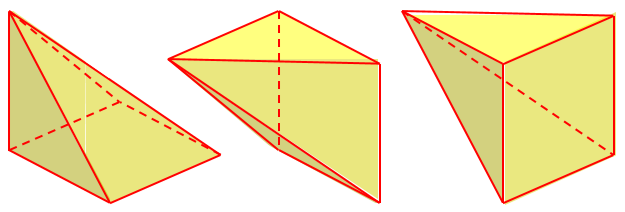You can make such a pyramid out of cardboard or index cards -- it's a nice project.  The hardest part is to find the shape of the two diagonal sides. Make three of the pyramids.

You'll find (maybe you can visualize this without actually making the pyramids) that the three pyramids can be put together to make one whole cube! Put the three "pointy ends" together, with the square bases of the other two pyramids vertical, one on the front right side and one on the back right side of the figure above.

Do you get it now? The volume of the cube is s*s*s if the sides are of length s. The volume of each of the identical pyramids must therefore be (1/3)s*s*s. The height (h) is s, and the area of the base (b) is s*s, so the area of this pyramid is (1/3)b*h.

Here is how they go together: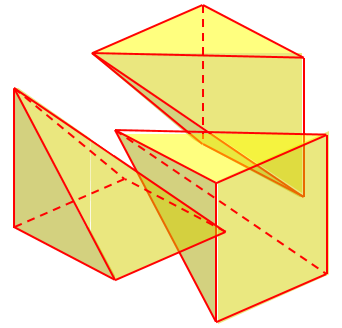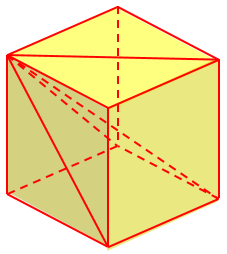Clearly the volume of each of these pyramids is $$\frac{1}{3}Bh = \frac{1}{3}s^3$$.

## Dissecting a cube into 6 pyramids

A 2005 question provides a somewhat different proof that may be easier to see:

Explanation of the Formula for the Volume of a Pyramid

OK, I know that the volume of a pyramid is Base*Height/3, but can you please, please explain why?  I couldn't find the answer on _why_ the formula works in your archives.

I know that a pyramid is basically a prism with some of it "shaved" off, so I guess this is why you have to divide Base*Height by 3.

Doctor Greenie referred to the first page we looked at, and commented,

On this page, there is a link to a site on the Internet containing a proof of this formula based on dividing a prism into 3 pyramids.  I myself find that figure hard to visualize, and the proof hard to follow.

This, of course, refers to Euclid’s proof. Like Doctor Rick, he gave as an alternative a special case rather than a general proof:

Here is a different approach which uses a similar process and a somewhat informal approach to argue that the formula is base times height divided by 3.

Suppose we start with a cube, and from the center of the cube we draw lines to the 8 corners of the cube.  This divides the cube into 6 congruent pyramids with square bases.

If the side of the cube is s, then the volume of the cube is s^3.

Here is the cube divided: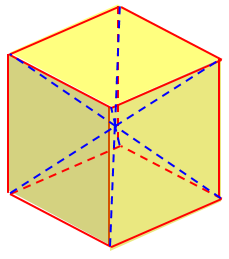In each of the pyramids, the base is a square of side s, and the height is half of s.  If we were to multiply the area of the base times the height to get the volume of each pyramid, then we would find that the volume of each pyramid is

(s^2)*(s/2) = (s^3)/2

But then the total volume of the 6 pyramids would be

6 * (s^3)/2 = 3s^3

But we know the total volume of the pyramids is the volume of the cube, which is s^3.

Here is one pyramid, with base s by s, and height $$\frac{s}{2}$$: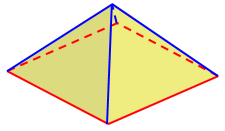Multiplying base times height to find the volume of a pyramid gives us a volume for the cube which is 3 times as large as it is supposed to be.  From this we can conclude that the volume of each pyramid is not base times height, but rather base times height divided by 3.

The volume of a prism containing this pyramid is $$s \cdot s\cdot \frac{s}{2} = \frac{s^3}{2}$$. The volume of the pyramid is $$\frac{1}{3}$$ of this.

Thanks so much for answering my question!  Your explanation was way better than the website you showed me.  I understand it better now.

Again, this was not a proof, but was convincing.

## Applying it to a non-cube

We’ll look at one last question (2016), in which we are not deriving a formula, but applying our thinking directly to a specific case:

Volume of a Square Pyramid by Construction, not Rote

I'm helping a student whose homework often calls for computing a quantity without resorting to a formula. Most of the time, I can come up with something. Not this time.

The geometry homework question was to compute the volume of a square pyramid without a formula. The square base is 10 units on a side. The pyramid height is also 10. The other two edges of the triangular faces were given as 12.2 units, and the height of each face is 11.2.

Using the formula? Easy-peasy.

Not using the formula? I'm stumped!

I did find a geometric construct for the volume of a square pyramid the height of which is 1/2 the length of the square base. You can stack six of these pyramids into a cube by joining their points, so the resulting volume is 1/6th of that of the constructed cube.

But you can't do that with a pyramid of equal height and square base sides.

I looked for a more general derivation of the formula, but didn't find one that was short enough to expect 10th graders to use in a homework assignment. I think there may be a trick construct for a cube with height equal to the length of its base; but I wasn't able to find it, and I'm sure not seeing it on my own.

Students in this class haven't even really had trig functions yet, let alone calculus.

It turns out that the idea behind your trick -- putting a bunch of congruent pyramids together to form a cube -- can also be used to put a bunch of non-congruent pyramids together to form a rectangular prism.

Picture your 10x10x10 cube composed of six congruent square pyramids, each with a 10x10 base and a height of 5. Now stretch the cube in one direction to form a 10x10x20 rectangular prism. The two pyramids in that rectangular prism that have square bases have precisely the volumes you are trying to compute: they each have 10x10 square bases and a height of 10. After the stretch, the other four pyramids now have bases that are 20x10; and the height of each of them is still 5.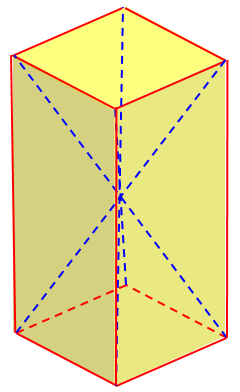Compared to the pyramids with the square bases, we have doubled one dimension of the base while halving the height, so the volumes are the same. Similarly, the six pyramids that make up the 10x10x20 rectangular prism still all have the same volumes.

The volume of the rectangular prism is 10*10*20 = 2000; and each of the six pyramids -- including the ones with 10x10 base and height 10 -- has the same volume. So the volume of your square pyramid with 10x10 base and height 10 is one-sixth of 2000, or 2000/6 = 1000/3.

Of course that is the answer that is obtained using the "easy-peasy" formula....

The reasoning here is much like Euclid’s way of showing his three pyramids have the same volume. Each has been doubled, by doubling one dimension.

### 2 thoughts on “Volume of a Pyramid – Without Calculus”

This site uses Akismet to reduce spam. Learn how your comment data is processed.